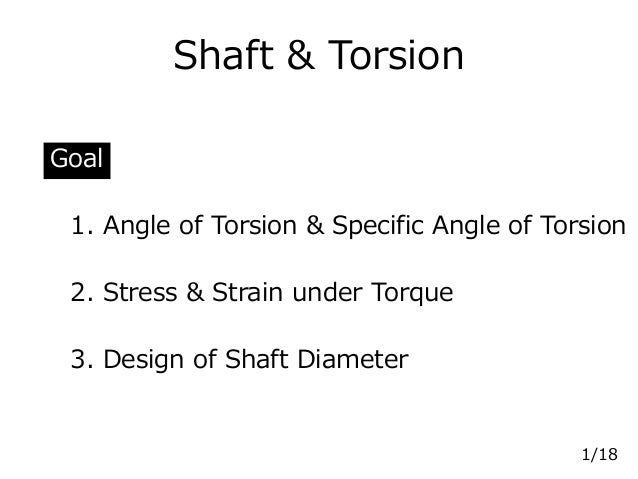Successfully reported this slideshow.Upcoming SlideShare
×

# Shaft & Torsion

2,049 views

Published on

Text book for the mechanics of materials

Shaft & Torsion
・Angle of Torsion & Specific Angle of Torsion
・Stress & Strain under Torque
・Design of Shaft Diameter

Published in: Engineering
• Full Name
Comment goes here.

Are you sure you want to Yes No• Be the first to comment

### Shaft & Torsion

1. 1. Shaft & Torsion 1. Angle of Torsion & Specific Angle of Torsion 2. Stress & Strain under Torque 3. Design of Shaft Diameter Goal 1/18
2. 2. Shaft & Torque & Torsion Shaft Torque Torsion：Twisting due to applied torque Torque：Moment around axis line [N・m]T Torsional moment 2/18 Axis line
3. 3. Machine Elements Subjected to Torque Ref. JAXA 3/18 Ref. waterstarmotors.clearmechanic.com
4. 4. Angle of Torsion Specific Angle of Torsion TA B B’ φ(x) θ ℓ x θ dφ(x) dx = ：Specific angle of torsionθ [ rad/m ] Angle of torsion per unit length r ：Angle of torsion [ rad ] θ Angle between two ends 4/18 Fixed
5. 5. Round Bar Uniform cross-section =ℓ θθ θ= θ ℓ 5/18 is constant in every cross-sectionθ
6. 6. Shear Deformation by Torsion a b c d a’ b’ c’ d’ γ a b c d a’ b’ c’ d’ AfterBefore 6/18Shear deformation on shaft surface No axial force
7. 7. Shear Strain A B B’ θ T γ ℓ r BB’ = γℓ = rθ γ= r ℓ θ = rθ 7/18 Fixed
8. 8. Shear Strain & Stress γ = rθ ℓ θ= r τ = G γ = Grθ ℓ θ= Gr r γ r τ ・angle of torsion ・radius Strain distribution Stress distribution Max. on surface 8/18 G：Shear modulus [Pa] ・length Proportional to Inverse proportional to
9. 9. Relationship between Torque and Shear Stress τ (ρ) ρ dρ r dA = 2πρdρ T T = A ρ・τ (ρ) dA τ (ρ)= τ r ρ = ρ dρ32π τr r 0 = 2 πr3 τ τ τ = πr3 2 T 9/18
10. 10. Polar moment of inertia of Area =Ip A ρ dA2 = r 0 ・2πρdρρ2 2 π r4 = ρ dρ dA = 2πρdρ 10/18
11. 11. Torsional Rigidity θ= T πr4 2 G = Ip T G 1 IpG : Torsional rigidity Torque to produce twist of 1[rad] Ip 2 π r4 = 2 π r4 1 T= 11/18 ∵ G
12. 12. Relationship with Torque 32 πd3 （ ）d: diameter τ = πr3 2 T γ = T= G τ πr3 2 G θ = r γ = T πr4 2 G T T πr3 32 G T πr4 64 G = r Ip T = r T IpG = Ip T G 1 12/18
13. 13. [Exercise] Stepped Round Bar θAB πr4 2 G ℓ1T 1 = θBC πr4 2 G ℓ2T 2 = θAB θBC＋θ = π 2 G T ℓ1 r4 1 ＋ ℓ2 r4 2 （ ）= T ℓ1 ℓ2 G A B C θ Left end is fixed T is applied at C Q. Angle of twist at right end ？ : Torque on cross-sectionT r1 r2 13/18 Fixed
14. 14. [Exercise] Allowable Stress & Shaft Diameter Allowable shear stress：τa Torque：T Q. Shaft diameter？ Max. shear stress Max. stress Allowable− < = πr3 2 Tτmax τ τa− < max r 3 πτa 2T−> 14/18
15. 15. Hollow Shaft If hollow r τ Stress is small in center ＝Bearing torque is small Not much decrease in capacity of bearing torque Decrease in mass 15/18 Stress distribution
16. 16. Shear Stress of Hollow Shaft Torque r rin T n= rin outr out = πr3 2 T τ （ ）n−1 4 out τ outr rin T = A ρ・τ (ρ) dA outr = ρ dρ32π τ rinoutr τ (ρ)= τ ρ outr = 2 outr π τ rin 44 −（ ）rout 16/18
17. 17. [Exercise] Solid vs. Hollow Round Shaft Allowable shear stress ：τa Torque：T Radius Mass (Vol.) Solid Hollow Hollow Solid 1.022 0.78 2.2 % increase 22 % decrease r 3= πτa 2T πr2 ℓV= V= n = 2 1 π ℓrin 22 −（ ）routoutr = 3 πτa 2T （ ）n−1 4 17/18
18. 18. Summary 1. Angle of Torsion, Specific Angle of Torsion 2. Stress & Strain under Torque 3. Design of Shaft Diameter ：Specific angle of Torsionθ [ rad/m ] Angle of torsion per unit length Allowable shear stress ：τa 18/18 ：Angle of Torsion [ rad ]θ Angle between two ends